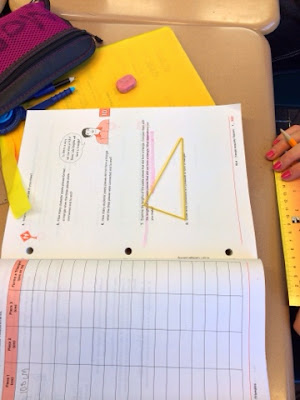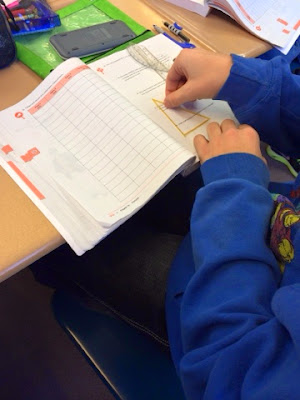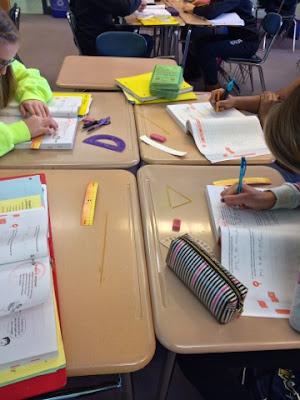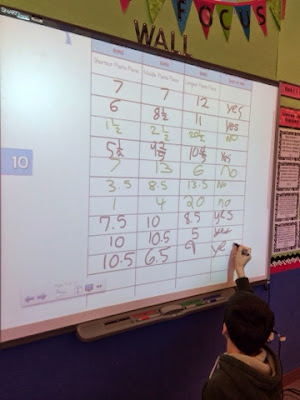## Tuesday, July 8, 2014

### Triangle Inequality Theorem- CCSS 7.G.2

Chapter 10 of Carnegie Learning Course 2 (7th Grade) is Triangles.  The lesson sequence for the chapter is shown below.
• 10.1 "Triangle Sum, Exterior Angle, and Exterior Angle Inequality Theorem
• 10.2 "Constructing Triangles"
• 10.3 "Congruent Figures and Constructing Congruent Triangles"
• 10.4 "Triangle Inequality Theorem"

I won't even get into it with you that geometry constructions tend to be the bane of my existence and that students are now doing constructions that were previously done in HS geometry.  That post if for another day.

I will tell you that I love this lesson and my students enjoyed it too.  It involves giving every student a piece of pasta and then having them randomly break it into 3 pieces.  The students then measure the three broken pieces (I had them do it in cm).

They put the three pieces together to see if they are able to form a triangle.  There is a table they fill out and then compare their results with their table group.We have a triangleAnother triangleStudents diligently workingBusy mathematicians dressed up for a spirit day
I then had students come up to the SMARTBoard to record their data.  I made sure to call on students who had/had not been able to form a triangle.  Students recorded the same data on that was on the SMARTBoard, other than the first data set was from their own findings.Sharing data with the whole class
This lesson does such a good job of building students conceptual understanding of the triangle inequality theorem.  They also are also able to figure out the triangle inequality theorem by looking at the data in the table.  They realize that the lengths of any two sides of a triangle must be greater than the third side.

The lessons ends with practice problems where students determine whether it's possible to form a triangle with a given set of side lengths and when given two side lengths, what can the conclude about the third length.

Prior to using Carnegie curriculum I used to model the triangle inequality theorem by cutting straws different lengths and then having student determine when they formed a triangle.

1.2.